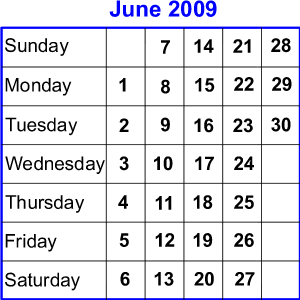#### You may also like### Prompt Cards

These two group activities use mathematical reasoning - one is numerical, one geometric.### Consecutive Numbers

An investigation involving adding and subtracting sets of consecutive numbers. Lots to find out, lots to explore.### Exploring Wild & Wonderful Number Patterns

EWWNP means Exploring Wild and Wonderful Number Patterns Created by Yourself! Investigate what happens if we create number patterns using some simple rules.

# Today's Date - 01/06/2009

##### Age 5 to 11Challenge LevelThis problem will be published for the first time on 1 June 2009, not an exciting date perhaps (even when written as $01$.$06$.$09$).

But in two days' time it will be $03.06.09$! Why do you think I might find that more exciting?

Earlier this year we had $8$ January $2009$ or $08$.$01$.$09$ ($8 + 1 = 9$).

There'll be some more like that this year. Can you find them?

Consider the following: $29$ March $1987$, or if you prefer $29$.$03$.$87$, or $3$.$29$.$87$
and then
$8$ November $1988$ or $8$.$11$.$88$

(Maybe one of these was the day someone you know was born.)

This time, $29 \times 3 = 87$ and $8 \times 11 = 88$.

We could also look at $27$ March $2009$ or $27$.$03$.$09$ as $27 \div 3 = 9$.
And we had the $18$ February or $18$.$02$.$09$ and that could be $18 \div 2 = 9$

All these may be called special 'productive' dates because we can find a mathematical relationship between the day, month and year.

I wonder which year, irrespective of the century, has the most 'productive' dates?

You could find all the calculations that work for the current year (if you are looking at this problem in years to come, it won't be 2009, but that doesn't matter!).